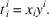International
Tables for
Crystallography
Volume D
Physical properties of crystals
Edited by A. Authier

International Tables for Crystallography (2006). Vol. D, ch. 1.1, p. 8

## Section 1.1.3.3.3. Contracted product, contraction

A. Authiera*

aInstitut de Minéralogie et de la Physique des Milieux Condensés, Bâtiment 7, 140 rue de Lourmel, 75015 Paris, France
Correspondence e-mail: aauthier@wanadoo.fr

#### 1.1.3.3.3. Contracted product, contraction

| top | pdf |

Here we are concerned with an operation that only exists in the case of tensors and that is very important because of its applications in physics. In practice, it is almost always the case that tensors enter into physics through the intermediary of a contracted product.

 (i) Contraction. Let us consider a tensor of rank 2 that is once covariant and once contravariant. Let us write its transformation in a change of coordinate system: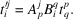Now consider the quantity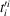derived by applying the Einstein convention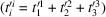. It follows that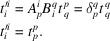This is an invariant quantity and so is a scalar. This operation can be carried out on any tensor of rank higher than or equal to two, provided that it is expressed in a form such that its components are (at least) once covariant and once contravariant. The contraction consists therefore of equalizing a covariant index and a contravariant index, and then in summing over this index. Let us take, for example, the tensor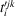. Its contracted form is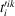, which, with a change of basis, becomes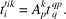The components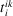are those of a vector, resulting from the contraction of the tensor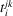. The rank of the tensor has changed from 3 to 1. In a general manner, the contraction reduces the rank of the tensor from n to. Example. Let us take again the operator of symmetry O. The trace of the associated matrix is equal to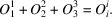It is the resultant of the contraction of the tensor O. It is a tensor of rank 0, which is a scalar and is invariant under a change of basis. (ii) Contracted product. Consider the product of two tensors of which one is contravariant at least once and the other covariant at least once: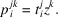If we contract the indices i and k, it follows that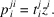The contracted product is then a tensor of rank 1 and not 3. It is an operation that is very frequent in practice. (iii) Scalar product. Next consider the tensor product of two vectors: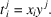After contraction, we get the scalar product: